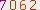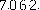Image.convert("1")

for x in range(0,image.size):

for y in range(0,image.size):

# print arr2[x][y]

if arr[x][y].tolist()==底色:

arr[x][y]=0

elif arr[x][y].tolist() in range(200,256) and arr[x][y].tolist() in range(200,256) and arr[x][y].tolist() in range(200,256):

arr[x][y]=0

elif arr[x][y].tolist()==[0,0,0]:

arr[x][y]=0

else:

arr[x][y]=255

arr是由numpy得到的,根据图片RGB值得出的矩阵,读者可以自己尝试完善代码，亲自实验一下。

IP代理池->左转Google右转baidu，有很多代理网站，虽然免费中能用的不多 但毕竟可以。